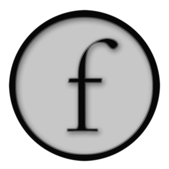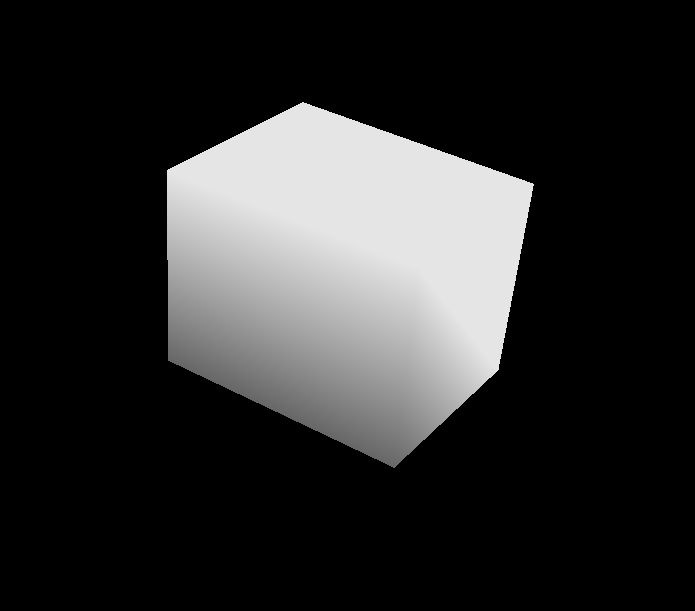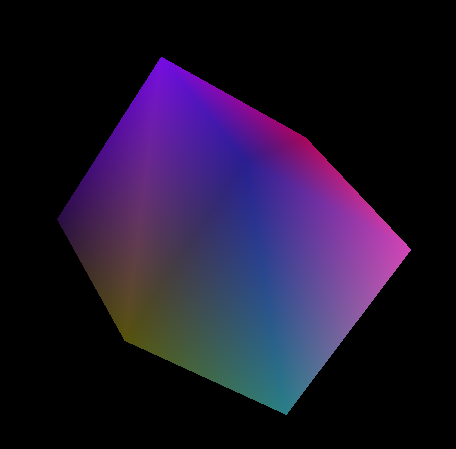# Loading FBX files into BaseGraphicsSystem

(This page assumes you've digested BaseGraphicsSystem and that you are familiar with Maya, 3ds Max or something like that).

Field's comes with a Java-based library for loading, and hacking the interesting parts out of, FBX files. FBX is a popular format for holding 3d geometry and animation data. All the major 3d products can read and write it. Now Field can read it as well.

OpenEnded Group uses FBX to read in assets from Maya and MotionBuilder — often assets that have come from motion capture. We've used this code quite a bit, but such is the sad complex lot of 3d graphics file formats that it's possible, perhaps likely, that you'll find a file that loads incorrectly. File a bug report and we'll see what we can do.

Currently, for dark reasons, Field's FBX interface no longer works under Java 1.5. Make sure you are running Field under 1.6 (see #201).

#### Tutorial

The FBX loading system is covered in this downloadable tutorial. This file includes an .fbx file to get you started.

### Summary

Since, if you are reading this page, you know something about 3d, here's the list of things that Field's FBX reader and graphics system can and can't do out of the box.

• Basic geometry — anything made out of triangles and quads. But nothing with arbitrary polygons. Hit it with a triangulate before exporting.
• Basic transform animation — anything that makes it out of FBX intact in terms of transform animations. Although we recommend against animating scale. Actually, we recommend against doing anything with the scale part of transforms at all.
• Basic skinning — Field will happily skin things, and animations the modify the transform tree will end up changing the skin of a character.
• Basic per-coordinate attributes — Field happily transports color, normal and texture coordinate data to OpenGL. What you actually do with that is up to your shaders. Note, Field doesn't support per-vertex-per-face data.

## Hello Cube

Let's make a cube in Maya:First of all, remember to triangulate it just to be on the safe side. Field (and most other real-time graphics systems) likes triangles and quads. But really, triangles are best.

And then "export all...":If you don't have Maya then use your favorite source of FBX data.

First, we need to turn on the jfbxlib plugin in the plugin manager.

Now, in Field we can write the following:

``````from field.extras.graphics import FieldJFBXExtensions
from field.extras.graphics.FieldJFBXExtensions import *

loader = loadFBX("/Users/marc/Desktop/aCube.fbx")
print loader
``````

This should print something like:

``````fbxLoader with 1 transforms, 1 meshes, 0 skinners
``````

`loader` now contains all of the things that Field knows how to load from an FBX file. We can begin to poke around:

#### getMeshes()

`getMeshes()` returns a `Map<String, TriangleMesh>` of all the meshes in the scene that you just loaded.

For example:

``````for meshName in loader.getMeshes():
print meshName
``````

Now that we've found some meshes — here the mesh 'pCube01' — the best thing to do is to put them onscreen. Recall from BaseGraphicsSystem:

``````from AdvancedGraphics import *

canvas = getFullscreenCanvas()
shader = makeShaderFromElement(_self)
canvas << shader
``````

This makes a new shader out of the current box, and sets it to be the default dark grey shader. Let's edit the `vertex_shader` slightly, to be just a little more exciting:

``````varying vec4 vertexColor;
attribute vec4 s_Color;
attribute vec3 s_Normal;

void main()
{
gl_Position = gl_ModelViewProjectionMatrix * gl_Vertex;
float light = abs(dot(s_Normal, vec3(-1.2,-0.4,0.9)));
vertexColor = vec4(light, light, light, 1.0);
}
``````

Don't forget to hit "refresh shader" on the toolbar of the text editor. All we've done is introduce some shading that's dependent on the vertex-normal.

Now we can go through all of the meshes that we loaded (well, all one of them):

``````for mesh in loader.getMeshes().values():
shader << mesh
``````

At this point you should see a white-ish cube on the screen:Remember you can drive the camera around, Doom style, using the keyboard.

## Hello animation

First of all let's just mention that we can animate this cube right now, directly in Field.

In a new box, we can type:

``````def spin():
transform = mesh.localTransform
# rotate around 'z' one complete turn
transform.r = Quaternion(Vector3(0,0,1), _t*Math.PI*2)
mesh.localTransform = transform

_r = spin
``````

Running this box, via option clicking or the timeline:gives us a box that spins as we move the mouse back and forth. Remember `_t` is a variable that goes from 0 to 1 as the mouse moves from the left to the right of the box. Hitting this box with a timeline gives you an animation that you can playback and scrub automatically.

Take a moment to look at SimpleLinearAlgebra to see some more `Vector3` and `Quaternion` things.

### More on transforms, part 1

Any mesh that you've loaded (indeed any mesh that you've created by any of the other means discussed in BaseGraphicsSystem) has one and exactly one transform associated with it. This transform is given to the shader, and appears as `gl_ModelViewProjectionMatrix`. Thus the first line of our shader `gl_Position = gl_ModelViewProjectionMatrix * gl_Vertex` transform says that the position of the vertex that we are actually going to draw is this transform matrix (and everything associated with the camera position and projection) multiplied by the 'local' position of the vertex.

This means that we can rotate, translate and scale this cube just by manipulating the transform leaving our original data intact. This is a non-destructive animation, and it's very fast. If that cube had 10000 vertices in it, rotating each on a little bit would start to get time consuming. Done this way, all of that transformation is actually done on the graphics card. The vertex data never "changes".

We'll see some more complex uses of transforms, including hierarchies of transforms in a moment.

## A more sophisticated example — a skinned humanoid

The FBX SDK from Autodesk comes with an example humanoid. You can download the whole thing here.

Sure enough, Field can load it:

``````loader = loadFBX("/Applications/Autodesk/FBXSDK20100/examples/ViewScene/humanoid.fbx")
for mesh in loader.getMeshes().values():
shader << mesh
``````

This yields a low-poly man:If you open it in Maya you'll find that that pose is not part of the animation. In fact it's the bind pose. This is the pose that the humanoid was skinned at.

The simplest way to start the animation is to call:

``````loader.applyAnimation(loader.getAnimationStart())
loader.updateSkinning()
``````

The `loader` contains animation data for a time period between `loader.getAnimationStart()` and `loader.getAnimationEnd()`. `.applyAnimation(time)` takes the animation data that's present in the FBX file, samples it at a particular time and dumps the results into all of the transforms that there's data for.

`.updateSkinning()` takes all of the transforms associated with any skinned object in the scene and updates all the skins accordingly.

Therefore to get a scrubbing animation, write this in a box:Here I'm using the time slider, rather than option clicking. Now we have a running man:### More on transforms, part 2

The animation that's in the file is an animation on a hierarchy of transforms that happen to puppet the skin around. This is the traditional way that smooth characters end up on screens today. If you are into modeling and animation you are undoubtedly familiar with this joint hierarchy.

#### `.getTransformRoots()`

The hierarchy is visible inside Field as well. To get the roots of this tree:

``````roots = loader.getTransformRoots()
print roots
``````

`roots` is a `Map<String, Element>`.

#### `.getTransformMap()`

To get all the transforms, use `.getTransformMap()`. So if you know the name of one of the coordinate system roots, you can look it up here:

``````transform = loader.getTransformMap()["Humanoid:RightLeg"]
local = transform.getLocal()
local.translation.x=0
transform.setLocal(local)
loader.updateSkinning()
``````

This random setting of the translation on a bone isn't normally the kind of thing you want to do to a human figure:Note that transforms in a hierarchy transform everything below them in the hierarchy as well. This makes perfect sense, when I rotate my elbow my wrist moves as well. Note also the call to `.updateSkinning()` so you can see the effects of your operation on the actual mesh.

## Raw Vertex data

One final note about the meshes themselves. A mesh in Field is 3 things: some vertices, some triangles (or lines or quads, but something that specifies the topology of the primitive that goes over the vertices) and optionally some more per-vertex information. The vertex data and the aux info get handed to the shader for the purposes of drawing.

How to get at this data? Let's go back to our cube example and start poking around the mesh:

#### `mesh.vertex()`

`mesh.vertex()` returns a `java.nio.FloatBuffer` with the vertex information associated with the mesh. Calling this function marks the mesh vertex data as 'dirty'. You have until the next frame is drawn to make your edits directly into this buffer.

The following code adds a little noise to the vertex data:

``````vertex = mesh.vertex()
for n in range(0, len(vertex)):
vertex[n] = vertex[n]+Math.random()*0.1-0.05
``````

If we just want to look at the vertex data directly, try:

``````vertex = mesh.vertex()
while vertex:
print Vector3(vertex)
``````

This prints, for my cube (your cube may vary):

``````    (-2.4516702, -1.9102246, 1.8331565)
(2.4005163, -1.8834419, 1.8353475)
(-2.3609655, 1.9182451, 1.866967)
(2.3689067, 1.8939898, 1.8179325)
(-2.4058237, 1.8717778, -1.856009)
(2.386585, 1.9141085, -1.8236033)
(-2.451188, -1.9386828, -1.8610132)
(2.3741024, -1.9233447, -1.8195552)
``````

#### `mesh.triangle()`

To look at the topology information use `mesh.triangle()`. It returns a `java.nio.ShortBuffer` with the triangles in it. They are grouped in threes and are indexes into the vertex data:

``````triangle = mesh.triangle()
while triangle:
print triangle.get(),triangle.get(),triangle.get()
``````

Prints:

``````    0 1 2
2 1 3
2 3 4
4 3 5
4 5 6
6 5 7
6 7 0
0 7 1
1 7 3
3 7 5
6 0 4
4 0 2
``````

If you have good powers of mental visualization you'll be able to convince yourself that by joining the vertices from the list above in the order given by the list below you get a cube. Certainly, if you count the number of things in the list you get the right number. 8 vertices, 12 triangles (for 6 faces).

You can also edit the topology buffer the same was as you edit the vertex data. Careful you don't ask for a vertex bigger than the number of vertices you actually have in the mesh. Graphics drivers really don't appreciate that.

#### `mesh.aux(id, size)`

Finally, to associate and edit arbitrary auxiliary data with vertices use the `mesh.aux(id, size)` method. `id` is a number from 1 to 15 (inclusive) and `size` is one of `1,2,3,4` and determines whether you are setting (in the parlance of GLSLang shaders) a `float`, a `vec2`, a `vec3` or a `vec4`.

For example, let's change our shader to:

``````varying vec4 vertexColor;
attribute vec4 s_Color;
attribute vec4 s_Five;

void main()
{
gl_Position = gl_ModelViewProjectionMatrix * gl_Vertex;
vertexColor = s_Five;
}
``````

and then call:

``````aux = mesh.aux(5, 4)
for n in range(0, aux.limit()):
aux.put(n, Math.random())
``````

Gives us:## Where next?

The FBX loader is completely production ready (although this page of documentation might not be). There are some tricks to getting marker animations and the like out of FBX files; connecting FBX cameras to Field cameras and for doing real geometry-cache vertex animations. Those will updated on demand.